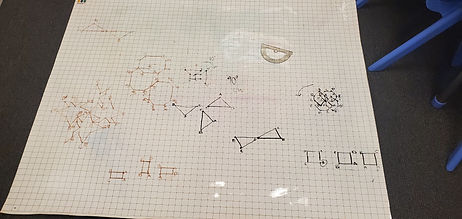## Mr. Jake

### Target 1​

###### Lesson Type:

New

Geometry

:

Symmetry

Graph symmetrical transformations on the coordinate plane.

###### 1:

Rotate a given figure around an origin in clockwise and counterclockwise directions and write the coordinates of the transformed image.

8th

###### Vocabulary:

Rotation, Coordinates, Vertex, Polygon

Activities:

Plotted shapes on the coordinate plane, choose a vertex of the shape, and rotated the shape around the vertex.### Home Exploration

###### Guiding Questions:## Absent Students:

### Target 2

:

###### 1:

Construct and read box and whisker plots.

###### 2:

Identify the parts of a box and whisker plot (median, quartile, upper quartile, lower quartile, and extremes).

###### 3:

Define median (the middle of a set of data), quartile (dividing data into four sections), upper quartile (the median of a quartile on the higher end of the range), lower quartile (the median of a quartile on the lower range), and extremes (the highest and lowest scores in the data range).

###### 4:

Draw a box and whisker plot to represent given data.

###### 5:

Order a set a data to find the median, quartiles, and extremes (minimum and maximum).

HS

###### Vocabulary:

Box and Whisker Plot, Median, Quartile, Minimum, Maximum

Activities:

Used a catapult to launch balls, collected data on how far they could launch the balls, and created box and whisker plots based off the data### Home Exploration

###### Guiding Questions:### Target 3

:

###### Vocabulary:

Activities:### Home Exploration